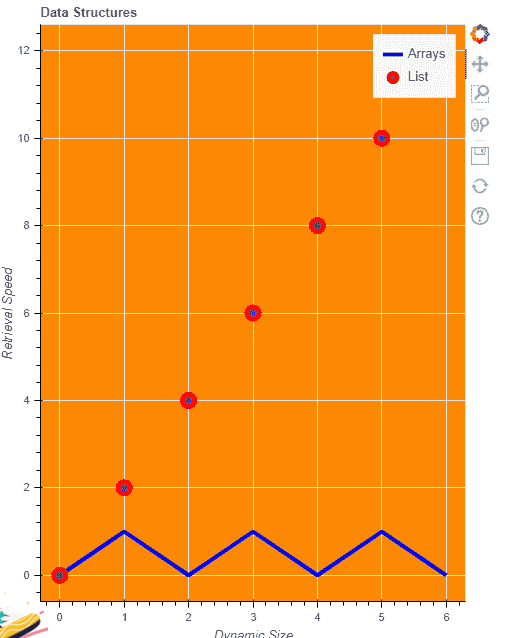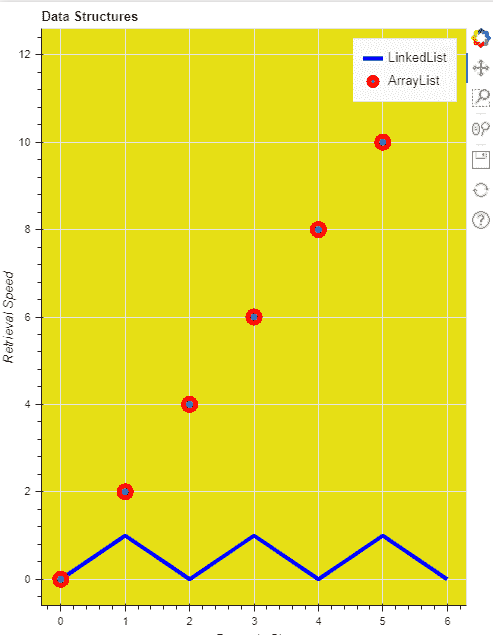﻿ 向博克图添加标签
﻿

# 向博克图添加标签

Bokeh 包括几个布局选项，用于排列图和小部件。它们可以安排多个组件来创建交互式仪表板或数据应用程序。布局功能允许您构建一个由图和小部件组成的网格。您可以将任意多的行、列或网格图嵌套在一起。此外，Bokeh 布局支持多种“尺寸模式”。这些调整模式允许绘图和小部件根据浏览器窗口调整大小。

## Python 3

``````# python program for adding label to pokeh plot
from bokeh.io import output_file, show
from bokeh.layouts import column
from bokeh.plotting import figure

# output will be in GFG.html
output_file("GFG.html")
currentList = list(range(7))

List2 = [i % 2 for i in currentList]
List3 = [i*2 for i in currentList]

f1 = figure(title="Data Structures",
x_axis_label="Dynamic Size",
y_axis_label="Retrieval Speed",
plot_width=500,
plot_height=650,
background_fill_color="#fc8803")

f1.line(currentList,
List2,
line_width=4,
line_color='blue',
legend_label='Arrays')
f1.circle(currentList,
List3, size=12,
line_width=5,
line_color='red',
legend_label='List')

show(f1)
``````## Python 3

``````# python program for giving label to plot
from bokeh.io import output_file, show
from bokeh.layouts import column
from bokeh.plotting import figure

# output will be in GFG.html
output_file("GFG.html")
currentList = list(range(7))

# creating two list from currentList
List2 = [i % 2 for i in currentList]
List3 = [i*2 for i in currentList]

# giving figure the basic attributes and labels
f1 = figure(title="Data Structures",
plot_width=500,
plot_height=650,
background_fill_color="#e6df15")

f1.xaxis.axis_label = "Dynamic Size"
f1.yaxis.axis_label = "Retrieval Speed"

# giving legend labels and other attributes
f1.line(currentList,
List2, line_width=4,
line_color='blue',

# giving legend labels and other attribute
f1.circle(currentList,
List3,
size=12,
line_width=5,
line_color='red',
legend_label='ArrayList')

# showing plots
show(f1)
``````﻿